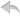注册    登录创作新主题

Python
 python开源   Django   Python   DjangoApp   pycharm
DATA
 docker   Elasticsearch

 问与答   闲聊   招聘   翻译   创业   分享发现   分享创造   求职   区块链   支付之战
WEB开发
 linux   MongoDB   Redis   DATABASE   NGINX   其他Web框架   web工具   zookeeper   tornado   NoSql   Bootstrap   js   peewee   Git   bottle   IE   MQ   Jquery

Python88.com
 反馈   公告   社区推广

Python社区  »  peewee

# PEEWEE 的数据库设计与实践

Py站长 • 5 年前 • 4736 次点击

## peewee 的入门

peewee 是一个非常精简的 Python ORM 库。它很容易上手，代码也很精简易读。快速上手 peewee 把 cookbook 都过一遍基本都没太大问题了。

peewee 的官方示例 app 基于 flask 。这或许是团队不熟悉的玩意。有兴趣的可以细看。

## one to one

one to one 实例的太直接太简单了。这里顺路提一下。在这个范例中，一个 user 归属于一个 group（的确这样不太实际，绝大部分应用都要求一个用户归属了多个组，这里就做个范例吧）。因此，group_id 代表用户的分组信息，直接放在 Users 表中。

show you the code.

```CREATE TABLE `users` (
`id` int(11) NOT NULL AUTO_INCREMENT,
`name` varchar(100) DEFAULT NULL,
`fullname` varchar(100) DEFAULT NULL,
PRIMARY KEY (`id`)
) ENGINE=MyISAM DEFAULT CHARSET=utf8;
INSERT INTO `users` VALUES (1,'otto',1),(2,'zjj',2);

CREATE TABLE `groups` (
`id` int(11) NOT NULL AUTO_INCREMENT,
`name` varchar(100) DEFAULT NULL,
PRIMARY KEY (`id`)
) ENGINE=MyISAM DEFAULT CHARSET=utf8
INSERT INTO `groups` VALUES (1,'oss'),(2,'dev');
```

peewee 中的 Model 定义：

```class Groups(BaseModel):
id = IntegerField()
name = CharField(null=True)

class Meta:
db_table = 'groups'

class Users(BaseModel):
id = IntegerField()
name = CharField()
group = ForeignKeyField(Groups, db_column='group_id')

class Meta:
db_table = 'users'
```

```for user in Users.select(Users.name, Groups.name).join(Groups):
print 'User (%s) in Group(%s)' % (user.name, user.group.name)
# output:
# User (otto) in Group(oss)
# User (zjj) in Group(dev)

SELECT t1.`name`, t2.`name`
FROM `users` AS t1
INNER JOIN `groups` AS t2
ON (t1.`group_id` = t2.`id`)
```

## one to one (no joining)

```CREATE TABLE `tasks` (
`id` int(11) NOT NULL AUTO_INCREMENT,
`name` varchar(100) DEFAULT '',
`content` varchar(100) DEFAULT '',
`type_id` int(11) default 0,
PRIMARY KEY (`id`)
) ENGINE=MyISAM DEFAULT CHARSET=utf8;

insert into tasks (`id`, `name`, `content`, `type_id`)
values
```

```select id, name, content from tasks where type = 0;
```

type 0 是啥？在哪里可以得知？项目代码里为了查验这个对应关系，在多个地方出现一个小 dict 来做翻译。代码可维护性就是这样降低的。即使良好一些的做法是在某个配置文件里写成一个 dict ，但是还是不尽如人意。

```class Tasks(BaseModel):
id = IntegerField()
name = CharField()
content = CharField()
type = IntegerField(db_column='type_id')

class Meta:

# output
# 0
# 1
```

```class TaskTypeField(Field):

# type_id 对应的 类型名称
type_dict = {
0: 'other',
1: 'finished',
2: 'waiting',
}
type_value_dict = { v:k for k, v in type_dict.iteritems() }

def db_value(self, value):
return self.type_value_dict.get(value, 0)

def python_value(self, value):
return self.type_dict.get(int(value), 'other')

id = IntegerField()
name = CharField()
content = CharField()

class Meta:
```

## one to many

one to many 的实现在于，把 one 的 id 放在每个 many 的个体中。

one to many 关键只是在于自动生成的 set 变量（如下的 messages_set）。

```class Users(BaseModel):
id = IntegerField()
name = CharField()

class Meta:
db_table = 'users'

class Messages(BaseModel):
id = IntegerField()
content = CharField()
user = ForeignKeyField(Users, db_column='user_id')

class Meta:
db_table = 'messages'

# 1
for user in Users.select(Users.name, Messages.content).join(Messages):
print user.name, user.messages.content

# 2
for user in Users.select(Users

.id, Users.name):
print user.name
for message in user.messages_set:
print '     ', message.content
```

## one to many(no joining)

```CREATE TABLE `tasks` (
`id` int(11) NOT NULL AUTO_INCREMENT,
`name` varchar(100) DEFAULT '',
`content` varchar(100) DEFAULT '',
`types` tinyint(11) DEFAULT 0,
PRIMARY KEY (`id`)
) ENGINE=MyISAM DEFAULT CHARSET=utf8

values
```

```class TaskTypeField(Field):

# 定义类型对应的位值
'other' : 2**0,
'useless' : 2**1,
'waiting' : 2**2,
'informed' : 2**3,
}

def db_value(self, value):
types_bit = 0
for type_name in value:
types_bit |= type_bit
return types_bit

def python_value(self, value):
types = []
if value & type_bit :
types.append(type_name)
return types

id = IntegerField()
name = CharField()
content = CharField()

class Meta:
```

```for task in Tasks.select(Tasks.id, Tasks.name, Tasks.types):
## output:
# ['other']
# ['useless', 'informed']
# ['useless', 'other', 'waiting']

```

## one to many(no multiple joining on the saume table)

```CREATE TABLE `principals` (
`id` int(11) NOT NULL AUTO_INCREMENT,
`name` varchar(100) DEFAULT NULL,
PRIMARY KEY (`id`)
) ENGINE=MyISAM DEFAULT CHARSET=utf8

CREATE TABLE `modules` (
`id` int(11) NOT NULL AUTO_INCREMENT,
`name` varchar(100) DEFAULT NULL,
`oss_principal1_id` int(11),
`oss_principal2_id` int(11),
`dev_principal1_id` int(11),
`dev_principal2_id` int(11),
PRIMARY KEY (`id`)
) ENGINE=MyISAM DEFAULT CHARSET=utf8
```

```class Principals(BaseModel):
id = IntegerField()
name = CharField()

class Meta:
db_table = 'principals'

OssPrincipal1 = Principals
OssPrincipal2 = Principals
DevPrincipal1 = Principals
DevPrincipal2 = Principals

class Modules(BaseModel):
id = IntegerField()
name = CharField()
oss_principal1 = ForeignKeyField(OssPrincipal1, db_column='oss_principal1_id')
oss_principal2 = ForeignKeyField(OssPrincipal2, db_column='oss_principal2_id')
dev_principal1 = ForeignKeyField(DevPrincipal1, db_column='dev_principal1_id')
dev_principal2 = ForeignKeyField(DevPrincipal2, db_column='dev_principal2_id')

class Meta:
db_table = 'modules'
```

```Modules.select(Modules, OssPrincipal1, OssPrincipal2) \
.join(OssPrincipal1)
.join(OssPrincipal2)
# ERROR:
_mysql_exceptions.OperationalError: (1066, "Not unique table/alias: 't2'")
```

```class Principals(BaseModel):
id = IntegerField()
name = CharField()

class Meta:
db_table = 'principals'

class PrincipalField(IntegerField):
principal_dict = {}
for principal in Principal.select().dicts():
principal_dict[principal['id']] = principal

def python_value(self,

_v):
principal_info = self.principal_dict.get(_v, {})
return principal_info

# def db_value(self, _v):
# not defined

class Modules(BaseModel):
id = IntegerField()
name = CharField()
oss_principal1 = PrincipalField(db_column='oss_principal1_id')
oss_principal2 = PrincipalField(db_column='oss_principal2_id')
dev_principal1 = PrincipalField(db_column='dev_principal1_id')
dev_principal2 = PrincipalField(db_column='dev_principal2_id')

class Meta:
db_table = 'modules'
```

```Modules.oss_principal1
# return { id: xx, name: }
```

## 树形结构树

```CREATE TABLE `products` (
`id` int(11) NOT NULL AUTO_INCREMENT,
`name` varchar(100) DEFAULT '',
`level` tinyint(5) ,
`parent_id` int(11),
PRIMARY KEY (`id`)
) ENGINE=MyISAM DEFAULT CHARSET=utf8;
```

```select products.name, children.name
from products
left outer join products as children on products.id = children.parent_id
```

```class Products(BaseModel):
id = IntegerField()
name = CharField()
level = IntegerField()
parent_id = IntegerField()

class Meta:
db_table = 'products'

@classmethod
def tree(class_, level=-1):
''' 获取最大级别到 level 的产品树信息 '''
query = class_.select(class_)
if level > 0:
query = query.where(class_.level <= level)
products = prefetch(query)
# TODO
# 在此处进行希望的数据结构的拼装
for product in products:
print product.name, product.id, product.level, product.parent_id
```

```# 获取三级的产品信息树
product_tree = Products.tree(level=3)
```
Python社区是高质量的Python/Django开发社区•   1 楼 cdxfish    5 年前 Do you have any tip about how to integrate Peewee with Django without disable Admin feature?